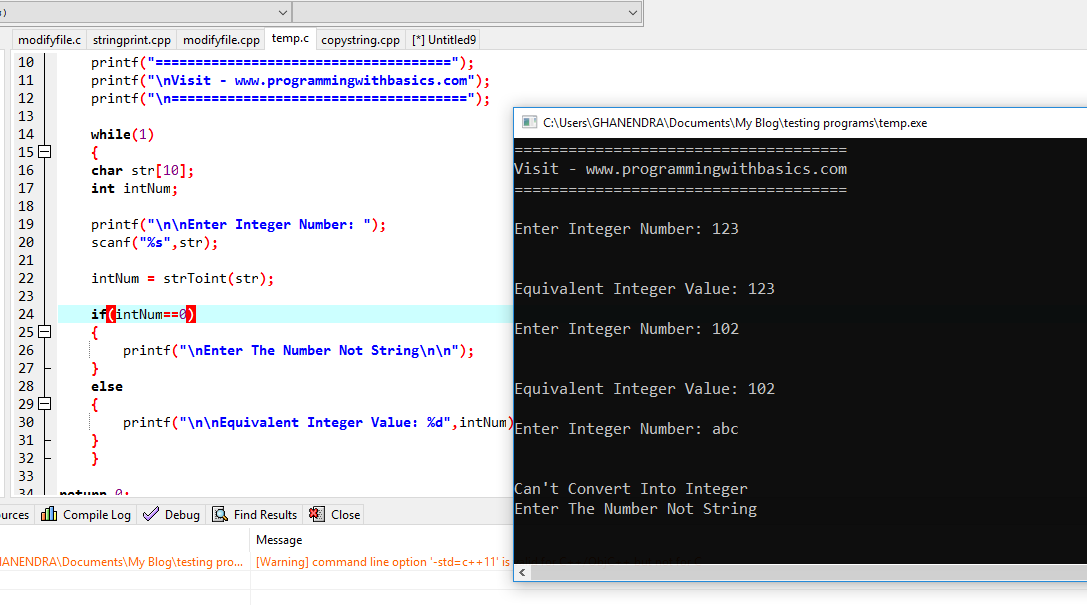# Convert String to Integer in C Without Using Atoi Function

Write a Program to Convert String to Integer in C Without Using Atoi Function. C string to an integer. how to convert string to int in c without Atoi. Here we are converting a string to an integer without using an Atoi library function, first, pass the string to a function and then compare it with the first if else condition.

If the condition is true then we can not convert a string into an integer and if the condition is false then we can convert a string to an integer number. Here we took an input number as a string, not as an integer that is why we have to convert the number to an integer or if we input the string then we cannot convert it.

## Convert String to Integer in C Without Using Atoi Function

``````#include <stdio.h>
#include <string.h>

int strToint(char[]);

int main()
{
/*Convert String to Integer in C Without Using Atoi Function*/

while (1)
{
char str;
int intNum;

printf("\nEnter Integer Number: ");
scanf("%s", str);

intNum = strToint(str);

if (intNum == 0)
{
printf("\nEnter The Number Not String\n\n");
}
else
{
printf("\nEquivalent Integer Value: %d", intNum);
}
}

return 0;
}

int strToint(char str[])
{
int i = 0, sum = 0;

while (str[i] != '\0')
{
if (str[i] < 48 || str[i] > 57)
{
printf("\nCan't Convert Into Integer");
return 0;
}
else
{
sum = sum *10 + (str[i] - 48);
i++;
}
}

return sum;

}``````

### The Output of Convert String to Integer in C### Similar to Convert String to Integer

Previous Post
Next Post

#### post written by: Ghanendra Yadav

Hi, I’m Ghanendra Yadav, SEO Expert, Professional Blogger, Programmer, and UI Developer. Get a Solution of More Than 500+ Programming Problems, and Practice All Programs in C, C++, and Java Languages. Get a Competitive Website Solution also Ie. Hackerrank Solutions and Geeksforgeeks Solutions. If You Are Interested to Learn a C Programming Language and You Don't Have Experience in Any Programming, You Should Start with a C Programming Language, Read: List of Format Specifiers in C.